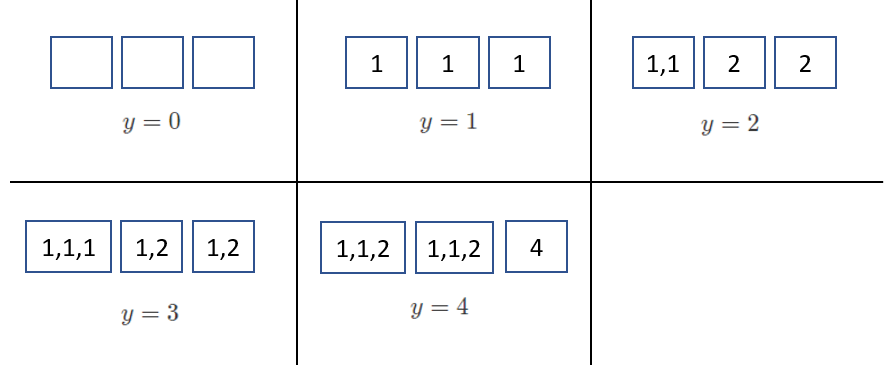# #567. 【IOI2020】Biscuits

Khong 阿姨在组织一场有 $x$ 位选手参加的竞赛，她打算给每位选手一袋饼干。总共有 $k$ 种不同类型的饼干，编号为从 $0$ 到 $k - 1$。类型为 $i$（$0 \le i \le k - 1$）的每块饼干都有一个口味值 $2^i$。在 Khong 阿姨的食品储藏室里，有 $a_i$（有可能为 $0$）块类型为 $i$ 的饼干。

### 实现细节

long long count_tastiness(long long x, std::vector<long long> a)
• $x$：需要装的饼干袋的数量。
• $a$：长度为 $k$ 的数组。对 $0 \le i \le k - 1$，$a_i$ 表示在食物储藏室里类型为 $i$ 的饼干数量。
• 此函数应当返回不同 $y$ 值的数目，使得阿姨可以装出 $x$ 袋饼干，且每袋饼干的总口味值都为 $y$。
• 此函数会被调用 $q$ 次（对于允许的 $q$ 值，详见约束条件和子任务部分）。每次调用应当被看成是独立的场景。

### 输入格式

• 第一行：$k\ x$
• 第二行：$a_0\ a_1\ \ldots\ a_{k - 1}$

### 输出格式

• 第 $i$ 行（$1 \le i \le q$）：count_tastiness 对于输入数据中第 $i$ 个场景的返回值。

### 样例一

#### input

2
3 3
5 2 1
3 2
2 1 2



#### output

5
6



#### explanation

count_tastiness(3, [5, 2, 1])

$y$ 能够取的值为 $[0,1,2,3,4]$。举例来说，为了装出总口味值均为 $3$ 的 $3$ 袋饼干，阿姨可以这样装：

• 一袋饼干里有 $3$ 块类型为 $0$ 的饼干，以及
• 两袋饼干，其中各有一块类型为 $0$ 的饼干和一块类型为 $1$ 的饼干。count_tastiness(2, [2, 1, 2])

$y$ 能够取的值为 $[0,1,2,4,5,6]$，由于总共有 $6$ 个可能的 $y$ 值，函数应当返回 $6$。

### 限制与约定

• $1\le k\le 60$
• $1\le q\le 1000$
• $1\le x\le 10^{18}$
• $0\le a_i\le 10^{18}$（对于所有的 $0\le i\le k-1$）
• 对于 count_tastiness 的每次调用，食物储藏室里所有饼干的口味值总和都不会超过 $10^{18}$。

$1$ $q\le 10$，且对于 count_tastiness 的每次调用，食物储藏室里所有饼干的口味值总和都不会超过 $100\ 000$。 $9$
$2$ $x=1,q\le 10$ $12$
$3$ $x\le 10\ 000,q\le 10$ $21$
$4$ 对于 count_tastiness 的每次调用，正确的返回结果都不会超过 $200\ 000$。 $35$
$5$ 没有附加限制条件。 $23$